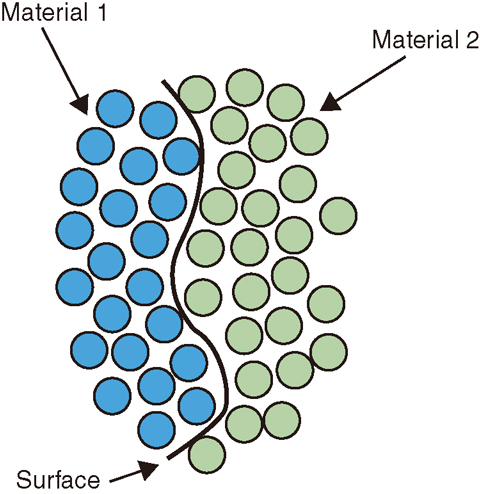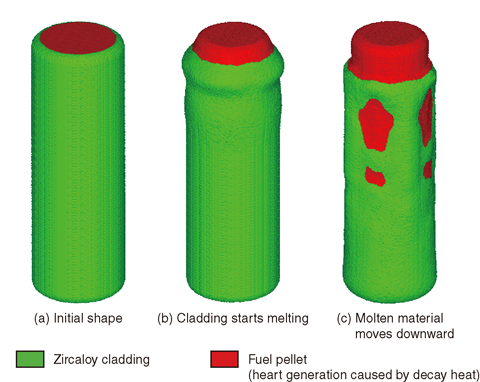# 1-15 Calculation of the Melting Behavior of Fuel Rods in Severe Accidents

## －Development of a Numerical Method Based on the Particle Method－Fig.1-35　Surface in the particle method

This figure shows a surface between different materials. Friction and surface tension forces act at the surface. A model that could calculate these forces was introduced to the POPCORN code, making it possible to calculate the interaction at the surface.Fig.1-36　Melting behavior of a fuel rod

These figures show the calculation results for the melting behavior of a fuel rod. (a) The fuel rod consists of zircaloy cladding and fuel pellets; (b) zircaloy cladding starts melting because of decay heat; (c) melting zircaloy relocates to the bottom, and UO2 remains in a solid phase because of the high melting temperature compared with the melting point of zircaloy.

Many numerical simulations are performed using severe accident codes for safety assessment of nuclear power plants. In severe accident codes, complex and diverse phenomena are simplified using the experimental results and numerical models because the calculation load is very high if these complex phenomena are calculated directly. Therefore, the accuracy of the calculation results is decreased in unexpected situations, because the models are not adequate to handle them. Experiments concerning severe accidents are not easy to perform because the experimental cost is very high and radioactive materials are difficult to use in such experiments. Therefore a detailed numerical simulation that can calculate a wider range of phenomena than severe accident codes plays an important role in evaluating the phenomena involved in a severe accident.

Thus, we are developing a numerical simulation code (POPCORN) for the melting behavior of fuel rods in severe accidents. In melting processes, it is expected that various types of phenomena will occur, including phase change, oxidation of zircaloy cladding and failure of fuel rods.To calculate the melting behavior of a fuel rod, we chose the particle method as a basis for developing the numerical code. In the particle method, fluid and solid materials are represented by groups of small particles, and not only fluid but also solid behavior can be calculated, with many types of materials being treated in a pressure vessel.

For the melting process, a large viscosity is set for molten materials to represent the fact that the molten materials have difficulty flowing, and interaction models are introduced to the POPCORN code at surfaces between different materials (Fig.1-35). Fig.1-36 shows the results of test calculations for the melting of fuel rods caused by decay heat. Zircaloy cladding melted because of decay heat, and molten zircaloy was relocated to bottom part.

In future work, chemical reactions and eutectic models will be introduced to the POPCORN code, and will be validated with experimental results.

| | | | |